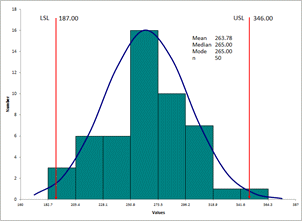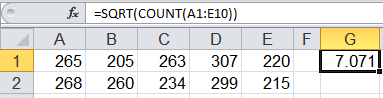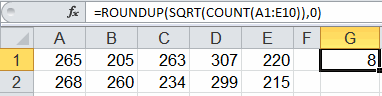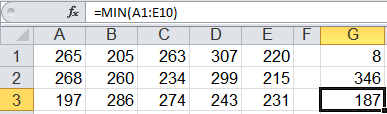# How to Determine Histogram Bin Width and Bin Intervals

## Histograms Are a Valuable Tool But They Can be Hard to Create

If you are creating a histogram manually or using Excel,
you will need to calculate:
the number of bins bin width bin intervals.The numerous steps are described below.

...or you can use QI Macros add-in for Excel.
you'll have a histogram drawn in seconds.### Here's How to Calculate the Number of Bins and the Bin Width for a Histogram

1. Count the number of data points.
2. Calculate the number of bins by taking the square root of the number of data points and round up.
3. Calculate the bin width by dividing the specification tolerance or range (USL-LSL or Max-Min value) by the # of bins.

### Let's Use an Example to Better Understand Bin and Bin Width Calculations

Description
Example of Calculation In Excel

Step 1: Count the Number of Data Points

Let's say we have 50 data points.

If your data is in Excel, use Excel's count function to determine the number of data points.Step 2: Calculate the # of Bins, then round up

# of bins = square root of the # of data points

the square root of 50 = 7.071, round up to 8Step 3: Calculate the Bin Width

Bin width = Spec Tolerance or Range ÷ number of bins

• Spec Tolerance = USL-LSL
• Range = Max - Min

If we use the range for this example:

Bin width = (Max-Min) ÷ Number of bins

Bin width = (346 - 187) ÷ 8 = 19.875### How to Determine Bin Intervals to Create a Histogram in Excel

Bin intervals need to span enough distance to include the upper and lower spec limits and the min and max values. Using the data in the previous example, follow these steps to determine bin intervals for a histogram:

1. select a beginning point that is lower than or equal to both the lower spec limit and the min value
2. calculate bin intervals in Excel by taking the beginning value + the bin width, + the bin width, etc.
3. round the calculated values if desired
4. select an ending point that is higher than or equal to both the upper spec limit and the max valueNote: Upper and lower specification limits are determined by the customer of the process. If you don't have spec limits, use the maximum and minimum values.

### Juran's Guidelines for Number of Bars

Juran's Quality Control Handbook provides these guidelines for the number of bars and states that they are not "rigid" and should be adjusted when necessary.

 Number of Data Points Number of Bars Number of Data Points Number of Bars 20-50 6 201-500 9 51-100 7 501-1000 10 101-200 8 1000+ 11-20

### Why Choose QI Macros to Perform Capability Analysis and Draw Histograms?### Affordable

• Only \$299 USD - less with quantity discounts
• No annual fees
• Free Technical Support### Easy to Use

• Works Right in Excel
• Calculates Cp Cpk Pp Ppk
• Accurate Results Without Worry### Proven and Trusted

• 100,000 Users in 80 Countries
• Celebrating 20th Anniversary
• Five Star CNET Rating - Virus Free